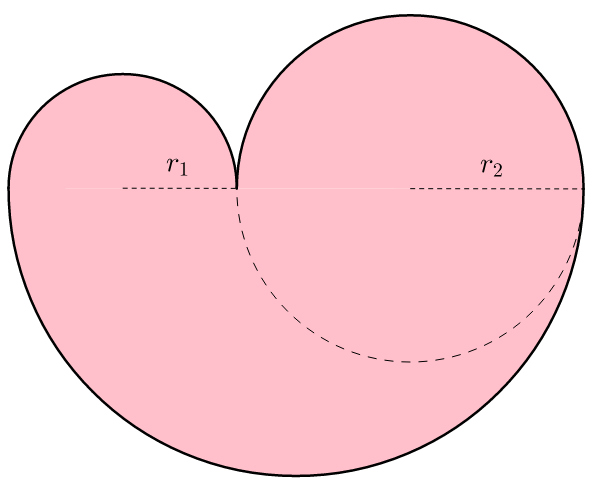# Me Want a Heart Limb!Mai Valentine planned to prepare a cake for Valentine's Day. While sketching out shapes and polygons, she thought about creating a heart shape, which resembles a combination of 3 semicircles of radii $r_1$, $r_2$ and $r_1 + r_2$, where $r_1 \leq r_2$. Joey Wheeler wants to take a limb as indicated by the dashed line. He claims that

After removing a limb, the new shape's area is half the original area of the heart.

What is the ratio of $r_2$ to $r_1$ that satisfies the given statement?

This is the end of the first chapter of the story. Check the following chapter directory if you are interested:

First - Second - Third - Fourth - Fifth

×